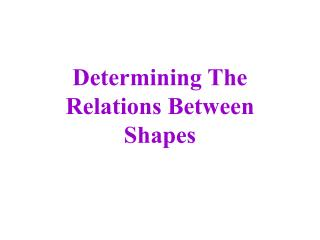DownloadDownload PresentationDetermining The Relations Between Shapes

# Determining The Relations Between Shapes

Download Presentation## Determining The Relations Between Shapes

- - - - - - - - - - - - - - - - - - - - - - - - - - - E N D - - - - - - - - - - - - - - - - - - - - - - - - - - -
##### Presentation Transcript

1. Determining The Relations Between Shapes

2. The 4 Geometric Shapes

3. HEXAGON • Six sides • It is a regular polygon • Equilateral (all sides are of equal length) • Equiangular (all interior angles are of equal measure)

4. RHOMBUS • Four sides • Equilateral (all sides are of equal length) • Opposite sides are parallel

5. TRAPEZOID • Four sides • One pair of opposite sides are parallel • Isosceles (two sides that are not parallel are of equal length)

6. TRIANGLE • Three sides • It is a regular polygon • Equilateral (all sides are of equal length) • Equiangular (all interior angles are of equal measure)

7. HEXAGON and RHOMBUS 1 3 1 2 1 hexagon = 3 rhombus

8. HEXAGON and TRAPEZOID 1 1 2 1 hexagon = 2 trapezoids

9. HEXAGON and TRIANGLE 1 2 3 1 6 5 4 1 hexagon = 6 triangles

10. RHOMBUS and TRAPEZOID 1/2 1 1 1 1/2 rhomus = 1 trapezoid

11. RHOMBUS and TRIANGLE 1 2 1 1 rhombus = 2 triangles

12. TRAPEZOID and TRIANGLE 1 1 2 3 1 trapezoid = 3 triangles

13. Determining the relations in some practice problems…

14. Independent Practice

15. If you finish early, you may go to the fun online activity that will give you some more practice with these shapes.http://arcytech.org/java/patterns/patterns_j.shtml